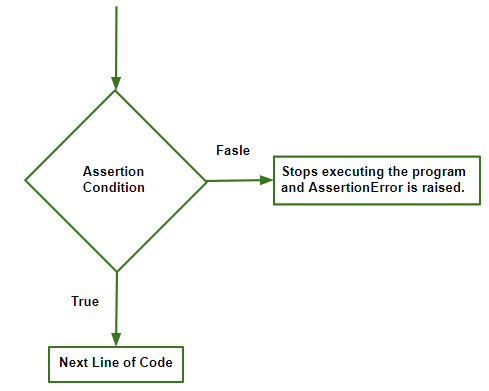# Python | Assertion Error

Assertion Error
Assertion is a programming concept used while writing a code where the user declares a condition to be true using assert statement prior to running the module. If the condition is True, the control simply moves to the next line of code. In case if it is False the program stops running and returns AssertionError Exception.
The function of assert statement is the same irrespective of the language in which it is implemented, it is a language-independent concept, only the syntax varies with the programming language.Syntax of assertion:
assert condition, error_message(optional)

Example 1: Assertion error with error_message.

 `# AssertionError with error_message. ` `x ``=` `1` `y ``=` `0` `assert` `y !``=` `0``, ``"Invalid Operation"` `# denominator can't be 0 ` `print``(x ``/` `y) `

Output :

```Traceback (most recent call last):
File "/home/bafc2f900d9791144fbf59f477cd4059.py", line 4, in
assert y!=0, "Invalid Operation" # denominator can't be 0
AssertionError: Invalid Operation

```

The default exception handler in python will print the error_message written by the programmer, or else will just handle the error without any message.
Both of the ways are valid.

Handling AssertionError exception:
AssertionError is inherited from Exception class, when this exception occurs and raises AssertionError there are two ways to handle, either the user handles it or the default exception handler.
In Example 1 we have seen how the default exception handler does the work.
Now let’s dig into handling it manually.

Example 2

 `# Handling it manually ` `try``: ` `    ``x ``=` `1` `    ``y ``=` `0` `    ``assert` `y !``=` `0``, ``"Invalid Operation"` `    ``print``(x ``/` `y) ` ` `  `# the errror_message provided by the user gets printed  ` `except` `AssertionError as msg:  ` `    ``print``(msg) `

Output :

```Invalid Operation
```

Practical applications.
Example 3: Testing a program.

 `# Roots of a quadratic equation ` `import` `math ` `def` `ShridharAcharya(a, b, c): ` `    ``try``: ` `        ``assert` `a !``=` `0``, ``"Not a quadratic equation as cofficient of x ^ 2 can't be 0"` `        ``D ``=` `(b ``*` `b ``-` `4` `*` `a``*``c) ` `        ``assert` `D>``=` `0``, ``"Roots are imaginary"` `        ``r1 ``=` `(``-``b ``+` `math.sqrt(D))``/``(``2` `*` `a) ` `        ``r2 ``=` `(``-``b ``-` `math.sqrt(D))``/``(``2` `*` `a) ` `        ``print``(``"Roots of the quadratic equation are :"``, r1, "", r2) ` `    ``except` `AssertionError as msg: ` `        ``print``(msg) ` `ShridharAcharya(``-``1``, ``5``, ``-``6``) ` `ShridharAcharya(``1``, ``1``, ``6``) ` `ShridharAcharya(``2``, ``12``, ``18``) `

Output :

```Roots of the quadratic equation are : 2.0  3.0
Roots are imaginary
Roots of the quadratic equation are : -3.0  -3.0
```

This is an example to show how this exception halts the execution of the program as soon as the assert condition is False.

Other useful applications :

• Checking values of parameters.
• Checking valid input/type.
• Detecting abuse of an interface by another programmer.
• Checking output of a function.

My Personal Notes arrow_drop_upCheck out this Author's contributed articles.

If you like GeeksforGeeks and would like to contribute, you can also write an article using contribute.geeksforgeeks.org or mail your article to contribute@geeksforgeeks.org. See your article appearing on the GeeksforGeeks main page and help other Geeks.

Please Improve this article if you find anything incorrect by clicking on the "Improve Article" button below.

Article Tags :

Be the First to upvote.

Please write to us at contribute@geeksforgeeks.org to report any issue with the above content.